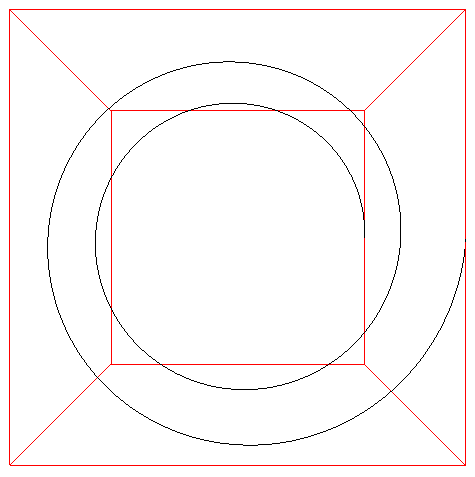# Horn

Graphics by Paul Bourne
March 2002
x = (2 + u cos(v)) sin(2 pi u)
y = (2 + u cos(v)) cos(2 pi u) + 2 u
z = u sin(v)
0 <= u <= 1, 0 <= v <= 2pi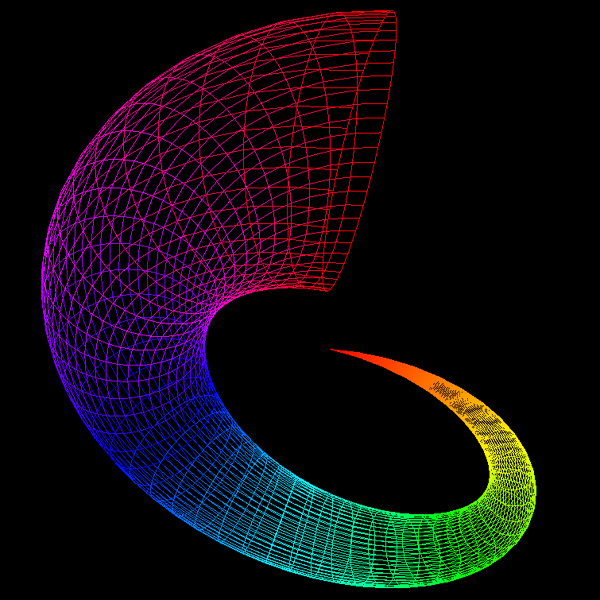# Crescent

By Paul Burke
August 2002
x = (2 + sin(2 pi u) sin(2 pi v)) sin(3 pi v)
y = (2 + sin(2 pi u) sin(2 pi v)) cos(3 pi v)
z = cos(2 pi u) sin(2 pi v) + 4 v - 2
0 <= u <= 1, 0 <= v <= 1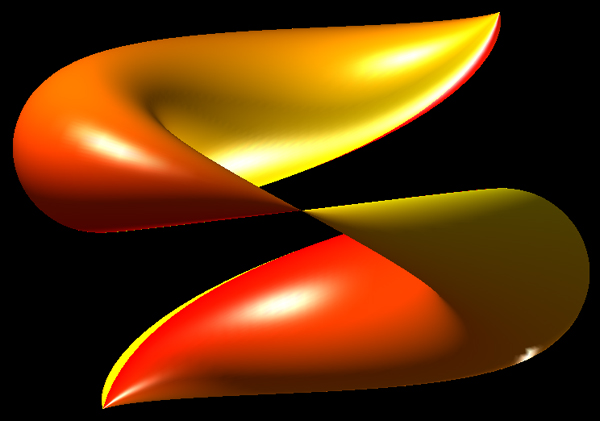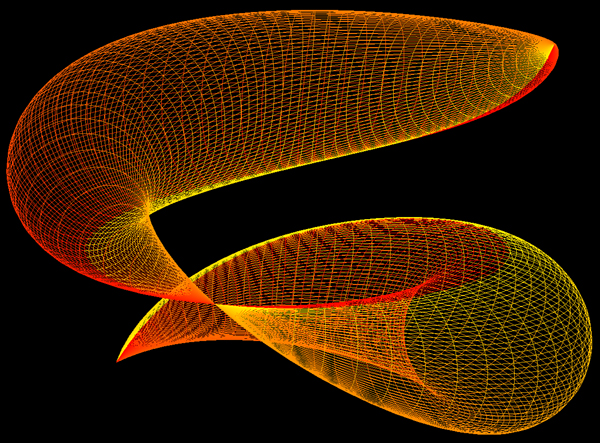# Mathematical Sea Shell

Created by Paul Bourke
May 1989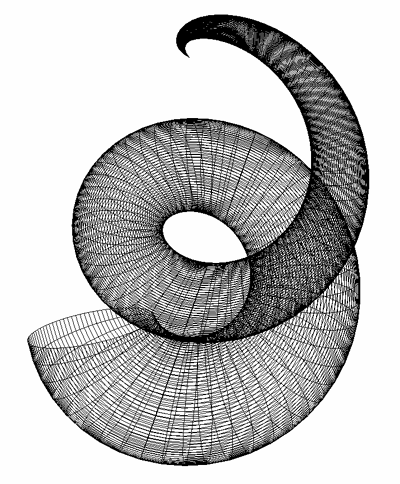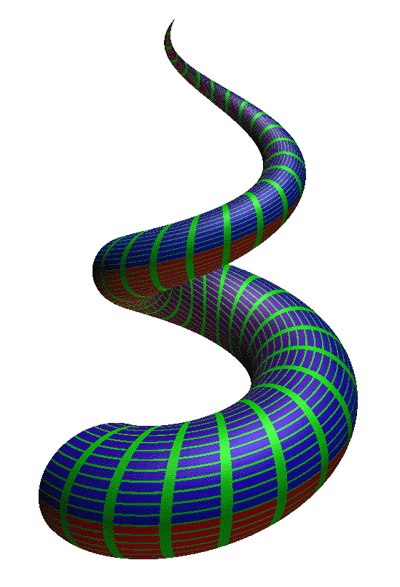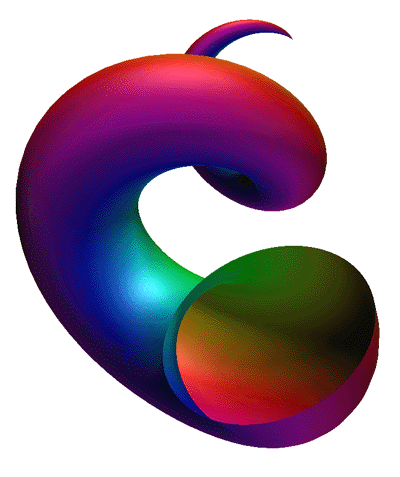# Modelling Springs

Written by Paul Bourke
November 2000

 r1 = 0.25, r2 = 0.25, periodlength=3.0, cycles = 4 Introduction This note describes one of many ways to describe a spring. The method used here is chosen because of the ease in which it can be used to describe a spring in a format suitable for computer modelling and rendering packages.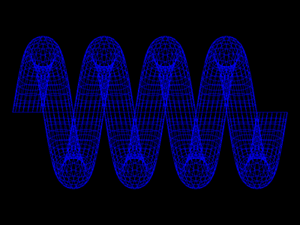r1 = 0.5, r2 = 0.5, periodlength=1.5, cycles = 3 Formula The formula below gives a point on the surface of a spring in Cartesian coordinates. It is a parametric equation in terms of parameters u and v. Both range from 0 to 2 pi, increasing u will give multiple windings. x = [1 - r1 * cos(v)] * cos(u) y = [1 - r1 * cos(v)] * sin(u) z = r2 * [sin(v) + periodlength * u / pi] Using these formula to create the 4 vertices of facets is fairly straightforward, see the C source code given below. Basically one evaluates the function for the parameters (u,v), (u+du,v), (u+du,v+dv), (u,v+dv) where du and dv are small increments, the exact amount depends on how smoothly one wants the surface to be represented.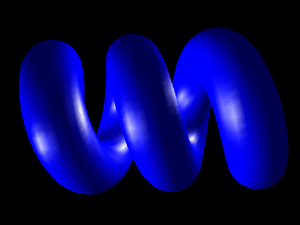r1 = 0.2, r2 = 0.2, periodlength=1.0, cycles = 3 Examples The examples on the right illustrate a small subset of the springs that can be created using the above formula. The parameters shown in red beside each example should make sense. For example, the number of cycles can be counted on the springs. The length of one winding is called the periodlength. The two radii determine the cross-section of the spring, circles for when r1 = r2 and squashed rings when r1 != r2.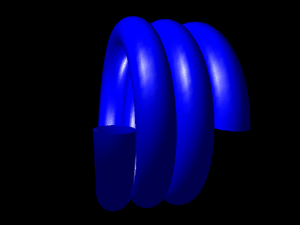r1 = 0.2, r2 = 0.5, periodlength=1.5, cycles = 3 Extensions Some exercises for the reader.....(1) this can easily be turned into a spring spiral by scaling the x and y values by some function of u. (2) Many rendering programs might insist on descriptions of solid objects in which case a cap will be needed on the ends of the spring. (3) The resolution of the facet approximation could be automatically determined by the number of cycles.r1 = 0.5, r2 = 0.2, periodlength=1.5, cycles = 5 Source code A simple piece of C code is given here: spring.c. It creates a faceted approximation to a spring including the calculation of unit normals for smooth shading. In this example the facets are written in geom format, it should be easy to modify the code to export in a format suited to your application. The images given here were rendered using OpenGL.Suggested improvement by Stefan Potrykus It is pointed out that a distortion occurs for large values of periodlength. The proposed correction for the value of z is as follows: z = r2 * sin(v) * sqr(1+(periodlength / pi)*(periodlength / pi)) + periodlength * u / pi Alternatively reformulated as follows x = [R - r * cos(v)] * cos(u) y = [R - r * cos(v)] * sin(u) z = r * sin(v) * sqr(1 + h2/R2) + h * u where h = periodlength / pi Or alternatively x = [R + r * cos(v)] * cos(u) + h * r * sin(v) * sin(u) / w y = [R + r * cos(v)] * sin(u) - h * r * sin(v) * cos(u) / w z = h * v + 2 * pi * R * r * sin(v) / w where w = sqrt((2 * pi * a)2 + h2)

# Dini's Surface or Twisted Pseudo-sphere

Written by Paul Bourke
October 1995

Surface of constant curvature (negative).
Named after Ulisse Dini

 x = a cos(u) sin(v) y = a sin(u) sin(v) z = a (cos(v) + log(tan(v/2))) + b u 0 <= u, 0 < v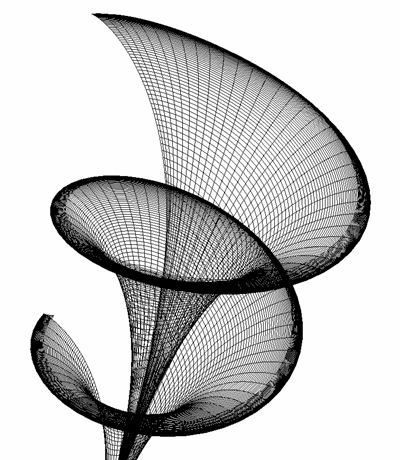Appeared on the cover of the Graduate Study in Mathematics, Western Kentucky University.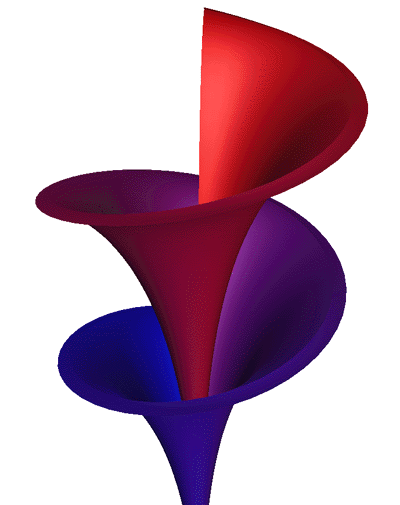Appeared on stickers for the MAA (Mathematical Association of America).

# Equiangular spiral

Also known as the logarithmic spiral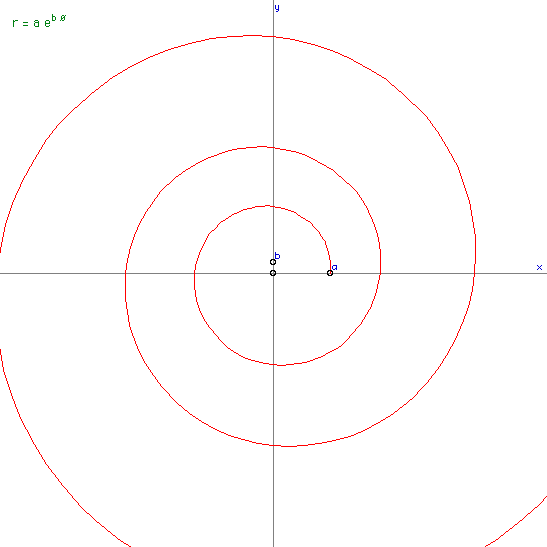# Fermat's spiral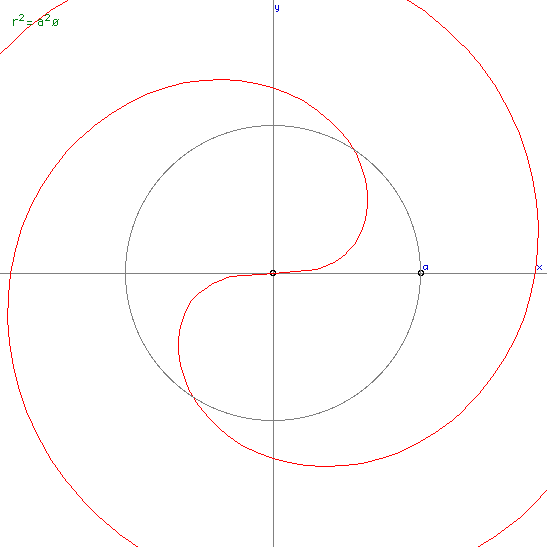# Parabolic spiral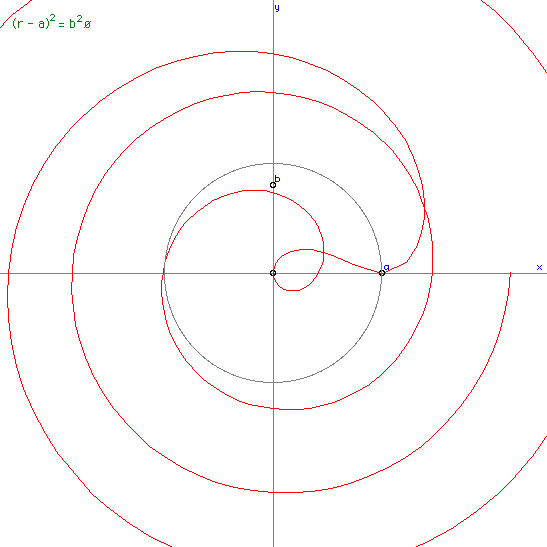# Hyperbolic spiral

Also known as the reciprocal spiral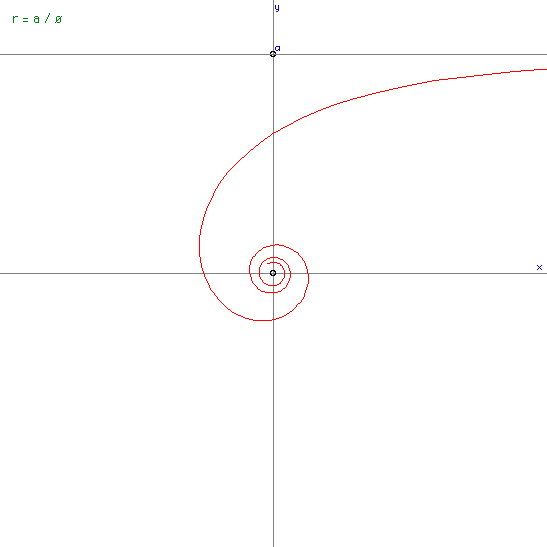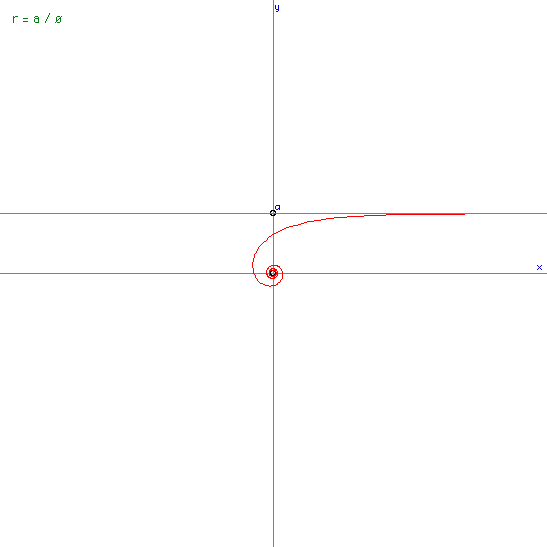# Lituus spiral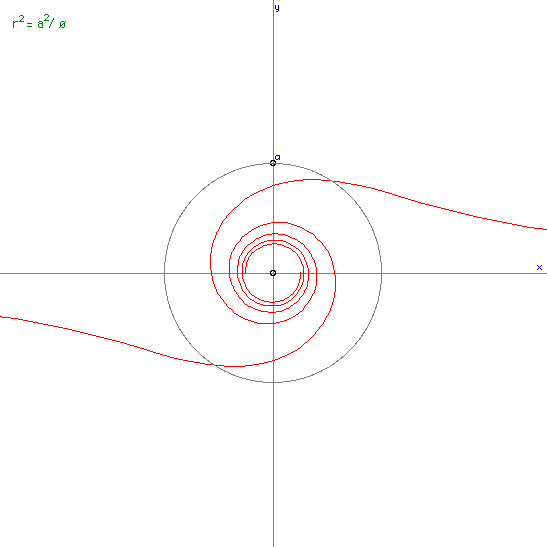# Sinusoidal spiral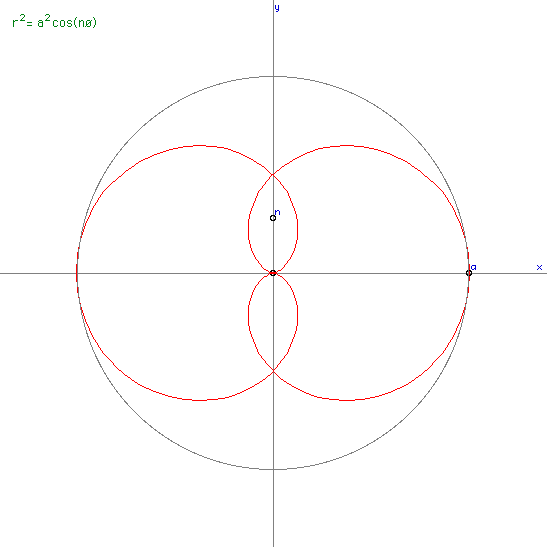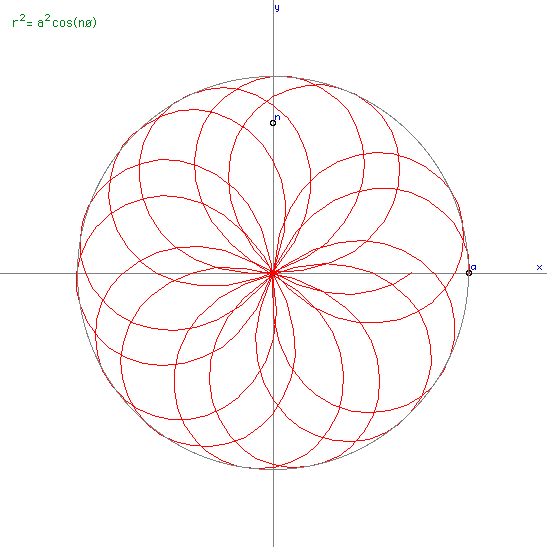# Square Archimedes spiral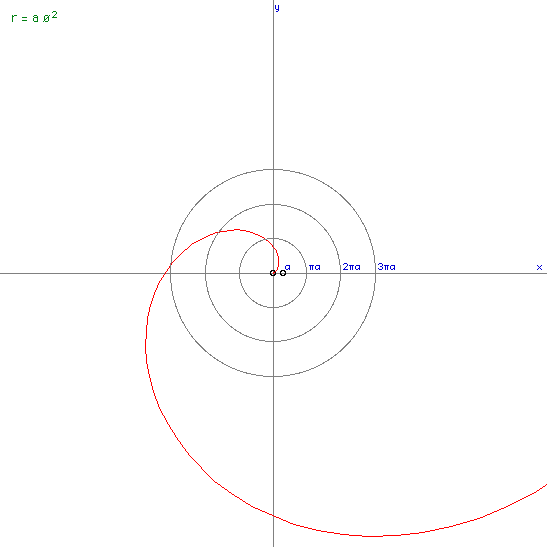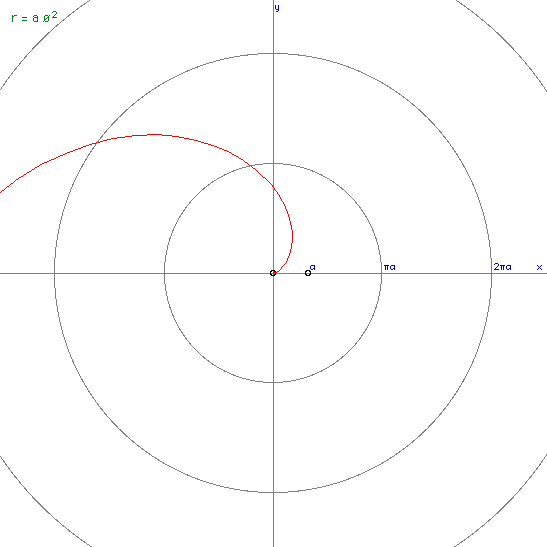# Cornu Spiral

Also known as Clothoid and Eulers Spiral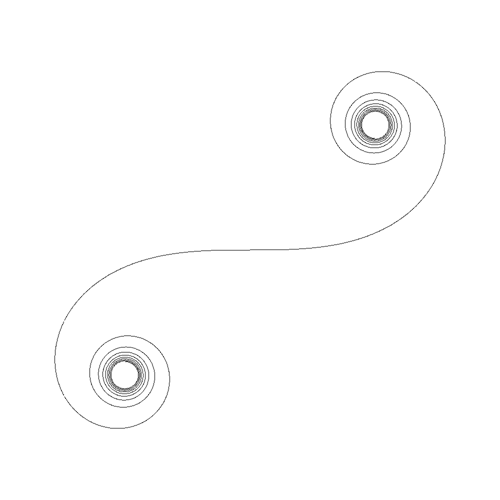The equation (a function of L) is given by the following, called the Fresnel integrals from their applications in optics.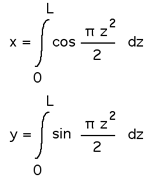These curves were most likely first studied Johann Bernoulli in the 1690's.

# Tanh Spiral

By Paul Bourke
January 2001
x = sinh(2 t) / (cos(2 a t) + cosh(2 t))
y = sin(2 a t) / (cos(2 a t) + cosh(2 t))
-pi/2 <= t <= pi/2, a > 0 (eg: 2)# Coth Spiral

By Paul Bourke
January 2001
x = -sinh(2 t) / (cos(2 a t) - cosh(2 t))
y = sin(2 a t) / (cos(2 a t) - cosh(2 t))
-pi/2 <= t <= pi/2, a > 0 (eg: 4)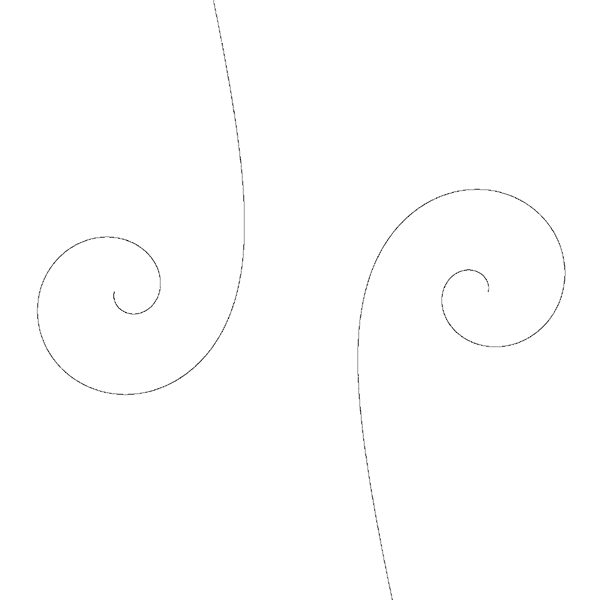# Helix

By Paul Bourke
January 2001
x = cos(t)
y = sin(t)
z = a t
0 < t < 4 pi, a = 0.2 (for example)